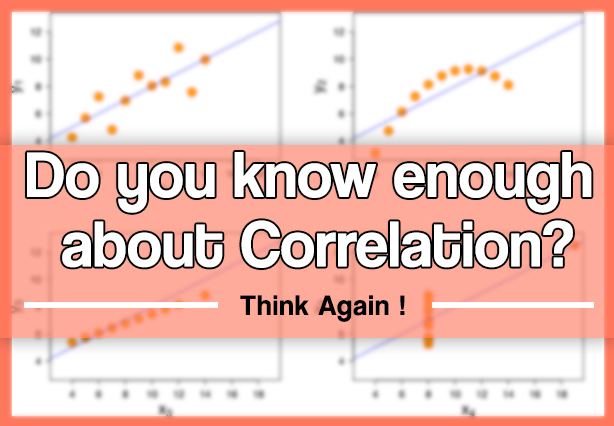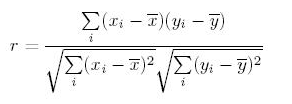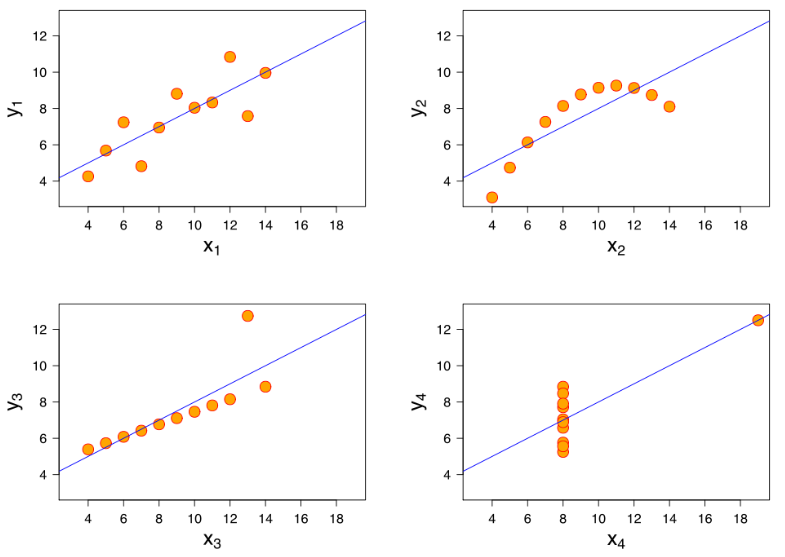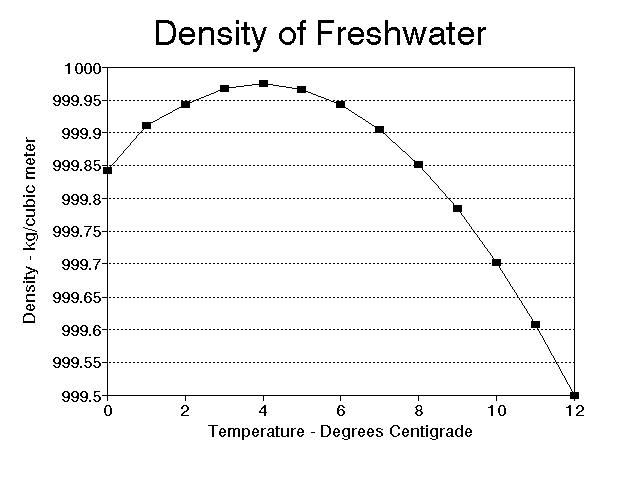Home » 7 most commonly asked questions on Correlation

# 7 most commonly asked questions on Correlation

## Introduction

The natural trajectory of learning statistics begins with measures of central tendency followed by correlation, regression to other advanced concepts. Amongst these initial concepts, I found correlation easy to understand, yet, got puzzled up when it got linked with other statistical concepts & metrices like causation, regression, distribution, pearson correlation coefficient etc. It took me sometime to succeed and get a firm hold on this concept. I succeeded because I kept on trying and tried harder, every time I failed. Hence, don’t settle, keep trying!

To begin with, if you are still struggling to understand the difference between correlation and causation, you should refer to my previous article where I’ve explained these concepts in the simplest possible manner.

Let’s proceed further and learn about the most commonly asked questions asked on correlation. If you are learning statistical concepts, you are bound to face these questions which mostly people try to avoid. For people like me, it should be a good refresher.

And if you’re looking to learn these questions for your data science interview, we are delighted to point you towards the ‘Ace Data Science Interviews‘ course! The course has tons of videos and hundreds of questions like these to make sure you’re well prepared for your next data science interview.## What you’ll learn ?

1. Does correlation and dependency mean the same thing? In simple words if two events have correlation of zero, does this convey they are not dependent and vice-versa?
2. If two variables have a high correlation with a third variable, does this convey they will also be highly correlated? Is it even possible that A and B are positively correlated to another variable C? Is it possible that A and B are negatively correlated with each other?
3. Can single outlier decrease or increase the correlation with a big magnitude? Is Pearson coefficient very sensitive to outliers?
4. Does causation imply correlation?
5. What’s the difference between correlation and simple linear regression?
6. How to choose between Pearson and Spearman correlation?
7. How would you explain the difference between correlation and covariance?
Answers to many of the above questions might seem intuitive, however you can find a few surprise factors in this article about correlation.

Let’s begin!

### Understanding the Mathematical formulation of Correlation coefficient

The most widely used correlation coefficient is Pearson Coefficient. Here is the mathematical formula to derive Pearson Coefficient.Explanation: It simply is the ratio of co-variance of two variables to a product of variance (of the variables). It takes a value between +1 and -1. An extreme value on both the side means they are strongly correlated with each other. A value of zero indicates a NIL correlation but not a non-dependence. You’ll understand this clearly in one of the following answers.

### Answer – 1: Correlation vs. Dependency

A non-dependency between two variable means a zero correlation. However the inverse is not true. A zero correlation can even have a perfect dependency. Here is an example :In this scenario, where the square of x is linearly dependent on y (the dependent variable), everything to the right of y axis is negative correlated and to left is positively correlated. So what will be the Pearson Correlation coefficient?

If you do the math, you will see a zero correlation between these two variables. What does that mean? For a pair of variables which are perfectly dependent on each other, can also give you a zero correlation.

Must remember tip: Correlation quantifies the linear dependence of two variables. It cannot capture non-linear relationship between two variables.

### Answer – 2: Is Correlation Transitive?

Suppose that X, Y, and Z are random variables. X and Y are positively correlated and Y and Z are likewise positively correlated. Does it follow that X and Z must be positively correlated?

As we shall see by example, the answer is (perhaps surprisingly) “No.” We may prove that if the correlations are sufficiently close to 1, then X and Z must be positively correlated.

Let’s assume C(x,y) is the correlation coefficient between x and y. Like wise we have C(x,z) and C(y,z). Here is an equation which comes from solving correlation equation mathematically :

C(x,y) = C(y,z) * C(z,x) - Square Root ( (1 - C(y,z)^2 ) *  (1 - C(z,x)^2 ) )

Now if we want C(x,y) to be more than zero , we basically want the RHS of above equation to be positive. Hence, you need to solve for :

 C(y,z) * C(z,x) > Square Root ( (1 - C(y,z)^2 ) *  (1 - C(z,x)^2 ) )

We can actually solve the above equation for both C(y,z) > 0 and C(y,z) < 0 together by squaring both sides. This will finally give the result as C(x,y) is a non zero number if following equation holds true:

C(y,z) ^ 2 + C(z,x) ^ 2 > 1

Wow, this is an equation for a circle. Hence the following plot will explain everything :If the two known correlation are in the A zone, the third correlation will be positive. If they lie in the B zone, the third correlation will be negative. Inside the circle, we cannot say anything about the relationship. A very interesting insight here is that even if C(y,z) and C(z,x) are 0.5, C(x,y) can actually also be negative.

### Answer – 3: Is Pearson coefficient sensitive to outliers?

The answer is Yes. Even a single outlier can change the direction of the coefficient. Here are a few cases, all of which have the same correlation coefficient of 0.81 :Consider the last two graphs(X 3Y3 and X 4Y4). X3Y3 is clearly a case of perfect correlation where a single outlier brings down the coefficient significantly. The last graph is complete opposite, the correlation coefficient becomes a high positive number because of a single outlier. Conclusively, this turns out to be the biggest concern with correlation coefficient, it is highly influenced by the outliers.

Check your potential: Should I become a Data Scientist?

### Answer – 4: Does causation imply correlation?

If you have read our above three answers, I am sure you will be able to answer this one. The answer is No, because causation can also lead to a non-linear relationship. Let’s understand how!

Below is the graph showing density of water from 0 to 12 degree Celsius. We know that density is an effect of changing temperature. But, density can reach its maximum value at 4 degree Celsius. Therefore, it will not be linearly correlated to the temperature.### Answer – 5: Difference between Correlation and Simple Linear Regression

These two are really close. So let’s start with a few things which are common for both.

• The square of Pearson’s correlation coefficient is the same as the one in simple linear regression
• Neither simple linear regression nor correlation answer questions of causality directly. This point is important, because I’ve met people thinking that simple regression can magically allow an inference that X causes. That’s preposterous belief.

What’s the difference between correlation and simple linear regression?

Now let’s think of few differences between the two. Simple linear regression gives much more information about the relationship than Pearson Correlation. Here are a few things which regression will give but correlation coefficient will not.

• The slope in  a linear regression gives the marginal change in output/target variable by changing the independent variable by unit distance. Correlation has no slope.
• The intercept in a linear regression gives the value of target variable if one of the input/independent variable is set zero. Correlation does not have this information.
• Linear regression can give you a prediction given all the input variables. Correlation analysis does not predict anything.

### Answer – 6: Pearson vs. Spearman

The simplest answer here is Pearson captures how linearly dependent are the two variables whereas Spearman captures the monotonic behavior of the relation between the variables.

For instance consider following relationship :

y = exp ( x )

Here you will find Pearson coefficient to be 0.25 but the Spearman coefficient to be 1. As a thumb rule, you should only begin with Spearman when you have some initial hypothesis of the relation being non-linear. Otherwise, we generally try Pearson first and if that is low, try Spearman. This way you know whether the variables are linearly related or just have a monotonic behavior.

### Answer – 7: Correlation vs. co-variance

If you skipped the mathematical formula of correlation at the start of this article, now is the time to revisit the same.

Correlation is simply the normalized co-variance with the standard deviation of both the factors. This is done to ensure we get a number between +1 and -1. Co-variance is very difficult to compare as it depends on the units of the two variable. It might come out to be the case that marks of student is more correlated to his toe nail in mili-meters than it is to his attendance rate.

This is just because of the difference in units of the second variable. Hence, we see a need to normalize this co-variance with some spread to make sure we compare apples with apples. This normalized number is known as the correlation.

### End Notes

Questions on correlation are very common in interviews. The key is to know that correlation is an estimate of linear dependence of the two variables. Correlation is transitive for a limited range of correlation pairs. It is also highly influenced by outliers. We learnt that neither Correlation imply Causation nor vice-versa.

### If you like what you just read & want to continue your analytics learning, subscribe to our emails, follow us on twitter or like our facebook page.

You can also read this article on our Mobile APP•tvmanikandan says:

Tavish,
I tried the following in R. I get pearson correlation as 0.88 and not 0.25 as you explained in the post. Please clarify.

> x=c(1:5)
> y=exp(x)
> y
 2.718282 7.389056 20.085537 54.598150 148.413159
> cor(x,y,method=”pearson”)
 0.8862751
> cor(x,y,method=”spearman”)
 1

•Tavish Srivastava says:

Try with
x <- c(1:100) you should get 0.25.

•Abhi says:

Very informative article! Thank you for writing this. If possible, could you also publish an article giving insights into various kinds of hypothesis testing techniques?

•Gaurav Kant Goel says:

Read this one and “difference between correlation and causation” back to back. My concepts on corelation have been solidified. Thanks for writing such informative articles.

•kalyanischakravarthi says:

Falling in love with “AV” . You guys are rocking.

•Nimesh Jha says:

Excellent Article . Thanks for writing in. it helped a lot.

•dunk says:

What’s about the sample size ?
Is there any rule of thumb regarding the sample size to measure correlation? I believe that different sample size can result in a different direction of correlation eg. N=100 correlation >0 but when N = 200 correlation <0

•M.Silambu says:

How to apply Correlation in retail business domain.could you please list some of the x and y values for retail business .Eg.total sales vs day of the week like that.